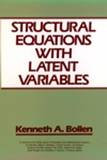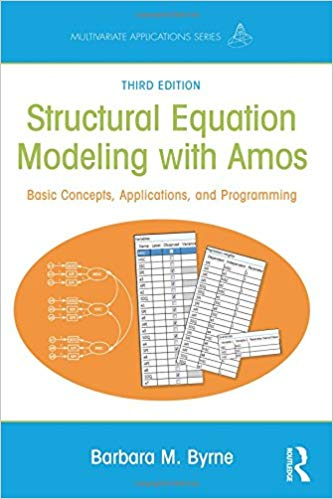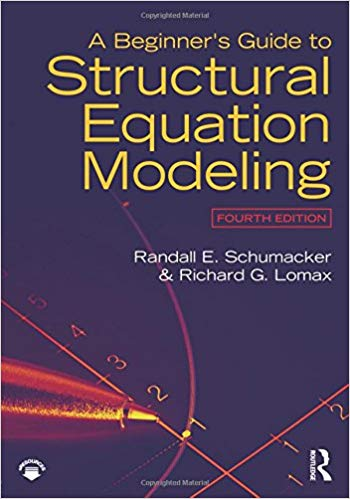## BooksKen Bollen, Structural Equations with Latent Variables . A comprehensive introduction to SEM models, including path analysis and CFABarbara Byrne, Structural Equation Modeling with AMOS A practical guide to structural equation modeling (SEM) using the Amos Graphical approach, 3rd Edition. If you use AMOS, this is sort of a how-to guide, organized to explain the basic ideas in CFA-SEM models. Data sets for the book: https://www.routledge.com/Structural-Equation-Modeling-With-AMOS-Basic-Concepts-Applications-and/Byrne/p/book/9781138797031#data-setsR. E. Schumacker & R. G. Lomax, A Beginner's Guide to Structural Equation Models . Noted for its crystal clear explanations, this book is considered the most comprehensive introductory text to structural equation modeling (SEM). Provides a 5 step approach to modeling data (specification, identification, estimation, testing, and modification).